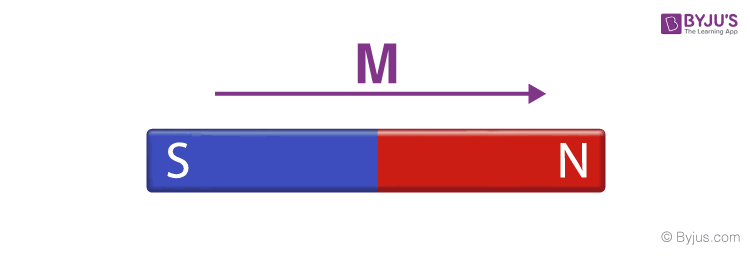# Magnetic Moment

Magnetic moment, also known as magnetic dipole moment, is the measure of the object’s tendency to align with a magnetic field.

“Magnetic Moment is defined as magnetic strength and orientation of a magnet or other object that produces a magnetic field.”

The magnetic moment is a vector quantity. The objects have a tendency to place themselves in such a way that the magnetic moment vector becomes parallel to the magnetic field lines.
The direction of the magnetic moment points from the south to the north pole of a magnet. The magnetic field created by a magnet is directly proportional to the magnetic moment.

### How is the magnetic moment produced?

Magnetic moment is generated by the following two methods:

• The motion of Electric Charge
• Spin Angular Momentum

### How is Magnetic Moment Measured?

Magnetic moments are typically measured by instruments known as magnetometers. But not all magnetometers are aligned to measure the magnetic moment directly. Some of these devices measure magnetic fields only and from the measured magnetic field, the magnetic moment is measured.

## Magnetic Moment Formula:

The magnetic moment is a vector relating torque of an object to the magnetic field. This is mathematically represented as:

 τ = m × B

Where,

• τ is the torque acting on the dipole
• m is the magnetic moment
• B is the external magnetic field

### Magnetic Moment Unit:

In the definition for the current loop, the Magnetic moment is the product of the current flowing and the area, M = I A

• So, the unit conferring to this definition is articulated by Amp-m2.
• It can also be suggested in terms of torque and moment. Conferring to that, the torque is measured in Joules (J) and the magnetic field is measured in tesla (T) and thus the unit is J T -1.
• So, these two units are equivalent to each other and are provided by 1 Amp-m2 = 1 J T -1.

Related Articles:

## What is Magnetic Dipole Moment?

A Magnetic Dipole comprises two unlike poles of equivalent strength and parted by a small distance.

For instance: The needle of a compass, a bar magnet, etc. are magnetic dipoles. We shall show that a current loop works as a magnetic dipole.

Magnetic Dipole Moment is described as the product of pole strength and the distance amidst the two poles. The distance between the two poles of a magnetic or a magnetic dipole is named as the magnet length and is given as the 2 ι.

If m is the power of any magnetic pole then the magnetic dipole moment of the magnet is signified by the vector M and it is represented asThe Magnetic dipole moment is a vector and it has a direction from the South Pole of the magnet to the north pole of the magnet, as presented in the figureMagnetic Dipole Moment

### Expression for Magnetic Dipole Force:

The force on a magnetic dipole is because of both the poles of the magnet, and we consider the magnetic dipole of a bar magnet and assume that the magnet is kept in an unbroken magnetic field B. In that situation, the force on the separate poles is articulated as

• mB which is along with the magnetic field B = Force on the N-pole
• mB and this is opposite to magnetic field B = Force on the S-pole

These forces are equivalent in magnitude, but opposite in direction and they form a parallel couple that rotates the magnet clockwise and creates a net torque on the magnet because of the individual force in a couple; thus we have torque acting on the bar magnet.

τ = Moment of the couple.

τ = mB × 2L sin θ

Where θ is the angle amid the magnet and the magnetic field, therefore, from the above discussion we have

M = m x 2L

Thus, Magnetic dipole moment is articulated by

ττ = MB sin θ

In vector form, it can be rephrased as:

 τ = M × B

This is the required expression for the magnetic dipole force.

## Magnetic Moment Questions

### What is the magnetic moment spin formula?

Following is the magnetic moment spin formula:

 $$\sqrt{n(n+2)}$$

Where n is the number of unpaired electrons.

### What is the magnetic moment of Mn2+ ion?

Mn2+ ion has 5 unpaired electrons. By using magnetic moment for spin formula $$\sqrt{n(n+2)}$$, where n is 5. We get the magnetic moment of Mn2+ as 5.96.

### What is the magnetic moment of Ni2+ ion?

Ni2+ ion has 2 unpaired electrons. By using magnetic moment for spin formula $$\sqrt{n(n+2)}$$, where n is 2. We get the magnetic moment of Ni2+ as 2.8.

### What is the magnetic moment of a bar magnet?

Consider a bar magnet of length l and with magnetic moment m. Bend the bar magnet in the form of a semicircular magnet, then the new magnetic moment will be:

 $$m’=\frac{2m}{\pi }$$

### What is the magnetic moment of electron?

An electron has a magnetic moment due to its orbital motion and is given as:

 $$\frac{\mu _{B}}{2 }$$

## Frequently Asked Questions – FAQs

### What is a Magnetic Moment?

Magnetic moment, also known as magnetic dipole moment, is the measure of the object’s tendency to align with a magnetic field.

### Is magnetic moment scalar or vector?

The magnetic moment is a vector quantity.

### How is magnetic moment produced?

The magnetic moment is produced by two methods: a) Motion of Electric Charge b) Spin Angular Momentum

### What is the magnetic dipole moment?

Magnetic Dipole Moment is described as the product of pole strength and the distance between the two poles.

### When is the magnetic moment zero?

In diamagnetic material, the electron orbits are random, and all the magnetic moments are cancelled. Similarly, all the spins are almost paired. Hence the net magnetic moment in the diamagnetic material is zero.